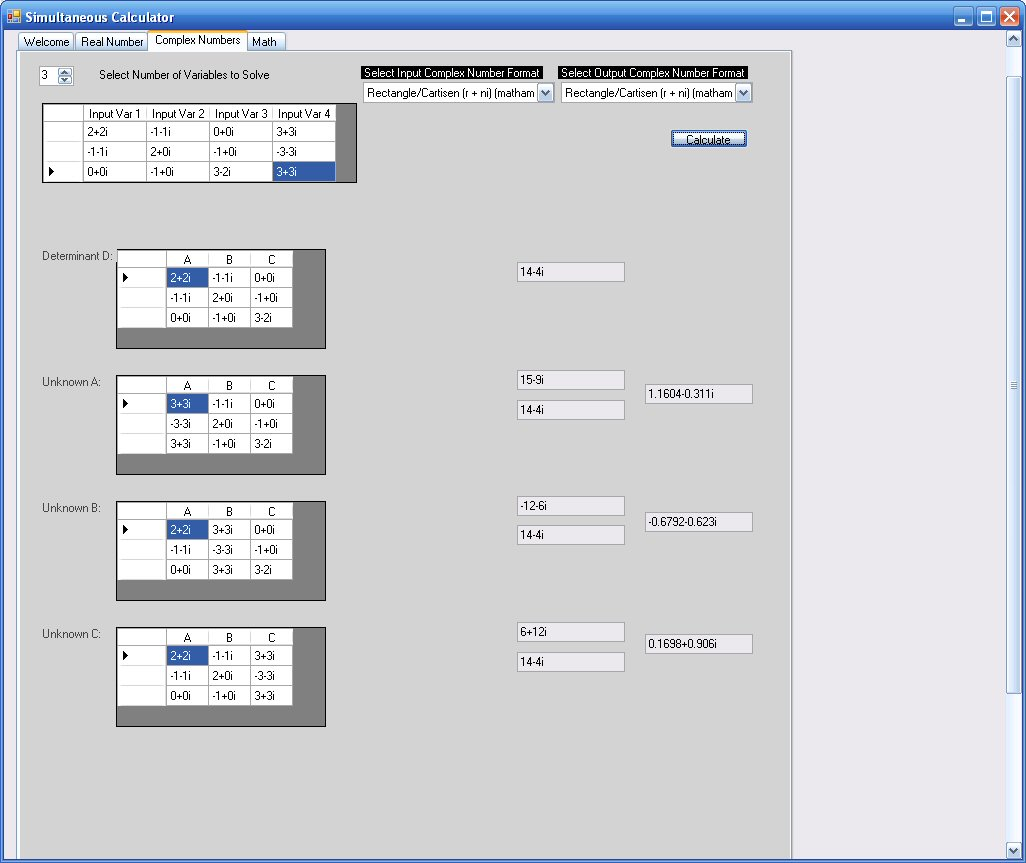Nov/28/2021

# Using Complex Numbers

This Simultaneous Calculator is a must for professionals as well as students in the engineering, scientific and mathmatic diciplins and field of studies.

This Simultaneous Calculator is a tremendous tool in solving simultaneous equations using complex numbers as well as real numbers.

This is a Windows PC base application that solves from 2 to 5 unknowns in either rectangle cartisian or polar formats. The rectangle form can be in either engineering or mathmitician format. Polar form can be represented in either angles or radians.

As well as solving the equations, this calculator provides a visual aid that demonstrates how the matrixes are form to calculate both the determinant as well as the matrics for the unknowns.

In addition, there is a format conversion tool and a more simple calculator that works with your complex numbers

This is an example of the Simultaneous Calculator's complex matrics for 3 unknown variables:We have two versions:

Student Version: \$9.95 - 5 month license for one semester.
Full Version: \$39.95 - one time purchase for current full function version.